# Knowing when to switch

How do you decide if a change you made to your webpage is getting more customers to sign up? How do you know if the new drug you invented cures more people than the current market leader? Did you make a groundbreaking scientific discovery?

All these questions can be answered using a branch of statistics called hypothesis testing. This post explains the basics of hypothesis testing.

The first question everyone has is: did it work? How do you know if what you are seeing is due to chance or skill? To answer this you need to know: how often would you declare victory just because of random variations in your data sample? Luckily you can choose this number! This is what p-values do for you.

But before diving into more details let's set up a little toy experiment to work with and illustrate the different concepts.

In :
%matplotlib inline

In :
import numpy as np
import matplotlib.pyplot as plt
import scipy as sp
import scipy.stats as stats
import random

In :
random.seed(12345)
np.random.seed(5345436)


The first thing we need is a set of observations. In this experiment you measured the conversion rate on your website. The average conversion rate is 6%. The first set of observations will always have an average conversion rate of 6%, using the difference parameter we can decide how big the difference between the two samples should be. This is handy as it allows us to generate a set of observations where the true difference is zero, or any other value we would like to investigate. We can also set how big the samples should be.

In :
def two_samples(difference, N=6500, delta_variance=0.):
As = np.random.normal(6., size=N)
Bs = np.random.normal(6. + difference, scale=1+delta_variance, size=N)
return As, Bs


What does this look like then? We will create two samples with the same mean and 100 observations in each.

In :
a = plt.axes()
As, Bs = two_samples(0., N=100)
_=a.hist(As, bins=30, range=(2,10), alpha=0.6)
_=a.hist(Bs, bins=30, range=(2,10), alpha=0.6)
print "Mean for sample A: %.3f and for sample B: %.3f"%(np.mean(As), np.mean(Bs))

Mean for sample A: 5.946 and for sample B: 6.093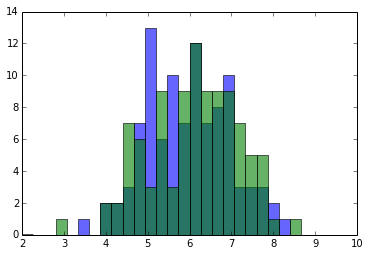You can see that the mean of neither of the two samples is exactly six, nor are the two values the same. Looking at the histogram of the two samples they do look kind of similar. If we did not know the truth about how these samples were made, would we conclude that they are different? If we did, would we be right?

This is where p-values and hypothesis testing come in. To do hypothesis testing you need two hypotheses which you would can pit against each other. The first one is called the Null hypothesis or $H_0$ and the other one is often referred to as "alternate" or $H_1$. It is important to remember that hypothesis testing can only answer the following question: should I abandon $H_0$?

In order to get started with your hypothesis testing you need to assume that $H_0$ is true, so the test can never tell you whether or not this assumption is a good one to make. All it can do is tell you that there is overwhelming evidence against your null hypothesis. It also does not tell you whether $H_1$ is true or not.

The p-value is often used (and abused) to decide if a result is "statistically significant". The p-value is nothing more than the probability that you observed a result as extreme (far away from $H_0$) or more extreme than the one you did by chance alone assuming that $H_0$ is true.

Let's stick with the example of us wanting to know if our changes to our website improved the conversion rate or not. The p-value is the probability for the mean in the second sample being bigger than the mean in the first sample due to nothing else but chance. In this case you can calculate the p-value by using Student's t-test. It is implemented in scipy so let's reveal it:

In :
def one_sided_ttest(A, B, equal_var=True):
t,p = stats.ttest_ind(A, B, equal_var=equal_var)
# the t-test implemented in scipy is two sided, but we are interested
# in the one sided p-value, hence this if statement and the divide by two.
if t < 0:
p /= 2.
else:
p = 1- p/2.
print "P-value: %.5f, the smaller the less likely it is that the means are the same"%(p)

one_sided_ttest(As, Bs)

P-value: 0.15576, the smaller the less likely it is that the means are the same


Common practice is to decide below which value the p-value has to be in order for this result to be statistically significant or not before looking at the data. By choosing a smaller value you are less likely to incorrectly conclude that your changes improved the conversion rate. Common choices are 0.05 or 0.01. Meaning you only make a mistake 1 in 20 or 1 in 100 times.

Let us repeat the experiment and look at another p-value:

In :
As2, Bs2 = two_samples(0., N=100)
one_sided_ttest(As2, Bs2)

P-value: 0.00285, the smaller the less likely it is that the means are the same


What happened here? The p-value is different! Not only is it different but it is also below 0.01, our changes worked! Actually we know that the two samples have the same mean, so how can this test be telling us that we found a statistically significant difference? This must be one of the cases where there is no difference but the p-value is small and we incorrectly conclude that there is a difference.

Let's repeat the experiment a few more times and keep track of all the p-values we see:

In :
def repeat_experiment(repeats=10000, diff=0.):
p_values = []
for i in xrange(repeats):
A,B = two_samples(diff, N=100)
t,p = stats.ttest_ind(A, B, equal_var=True)
if t < 0:
p /= 2.
else:
p = 1 - p/2.

p_values.append(p)
plt.hist(p_values, range=(0,1.), bins=20)
plt.axvspan(0., 0.1, facecolor="red", alpha=0.5)

repeat_experiment()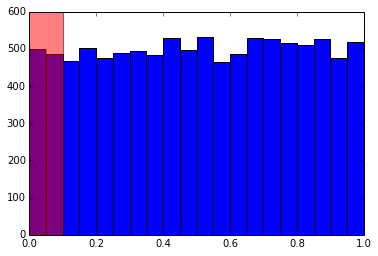The p-value depends on the outcome of your experiment, that is which particular values you have for your observations. Therefore it is different everytime you repeat the experiment. You can see that roughly 10% of all experiments ended up in the red shaded area, they have p-values below 0.1. These are the cases where you observe a significant difference in the means despite there being none. A false positive.

What happens if there is a difference between the means of the two samples?

In :
repeat_experiment(diff=0.05)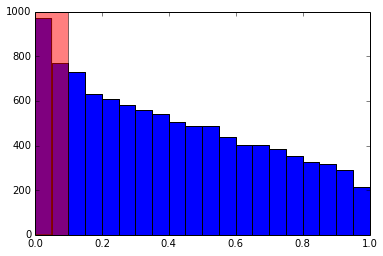Now you get a p-value less than 0.1 more often than 10% of the time. This is exactly what you would expect as the Null hypothesis is not true.

An important thing to realize is that by choosing your p-value threshold to be say 0.05, you are choosing to be wrong 1 in 20 times. Keep in mind: This is true if you judged a lot of copies of this experiment. For each individual experiment you do, you are either right or wrong. The trouble is you do not know which one of the two it is.

The smaller a value you choose for your p-value threshold, the smaller the chance of being wrong when you decide to switch to the new webpage. Nobody likes being wrong so why not always choose a very, very small threshold?

The price you pay for choosing a lower threshold is that you will end up missing out on opportunities to improve your conversion rate. By lowering the p-value threshold you will conclude that the new version did not improve things when it actually did.

In :
def keep_or_not(improvement, threshold=0.05, N=100, repeats=1000):
keep = 0
for i in xrange(repeats):
A,B = two_samples(improvement, N=N)
t,p = stats.ttest_ind(A, B, equal_var=True)
if t < 0:
p /= 2.
else:
p = 1 - p/2.

if p <= threshold:
keep += 1

return float(keep)/repeats

improvement = 0.05
thresholds = (0.01, 0.05, 0.1, 0.15, 0.2, 0.25)
for thresh in thresholds:
kept = keep_or_not(improvement, thresh)*100
plt.plot(thresh, kept, "bo")

plt.ylim((0, 45))
plt.xlim((0, thresholds[-1]*1.1))
plt.grid()
plt.xlabel("p-value threshold")
plt.ylabel("% cases correctly accepted")

Out:
<matplotlib.text.Text at 0x106ede550>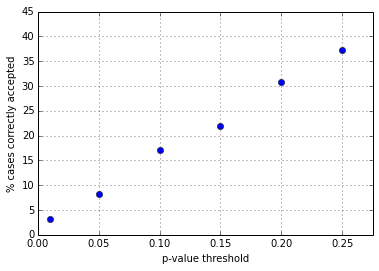From this you can see that the times you accept the new webpage (which we know to be better by 5%) is smaller if you choose your p-value lower. Missing out on these opportunities is the price you pay for being wrong less often.

For a fixed p-value threshold, you correctly decide to change your webpage more often if the effect is larger:

In :
improvements = np.linspace(0., 0.4, 9)
for improvement in improvements:
kept = keep_or_not(improvement)*100
plt.plot(improvement, kept, "bo")

plt.ylim((0, 100))
plt.xlim((0, improvements[-1]*1.1))
plt.grid()
plt.xlabel("Size of the improvement")
plt.ylabel("% cases correctly accepted")
plt.axhline(5)

Out:
<matplotlib.lines.Line2D at 0x10711b910>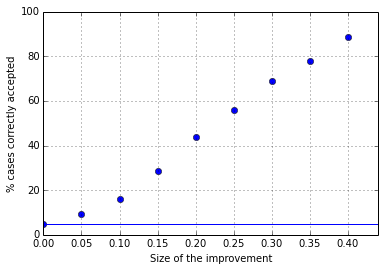This makes sense. If the difference between your two onversion rates is larger, then it should be easier to detect. As a result you correctly choose to change your webpage in a higher fraction of cases. In other words the larger the difference, the more often you correctly reject the Null hypothesis.

The horizontal blue line marks the p-value threshold of 5%. You can see for the left most point at 0% improvement, we reject the Null hypothesis in 5% of cases and change our webpage. In reality the new webpage does no better than what we had before.

Similarly, the larger your p-value threshold the more often you correctly decide to reject the Null hypothesis. This comes at a price though, because the larger your p-value threshold, the higher the chance of you incorrectly deciding to change the website.

What we have called "% cases correctly accepted" is known in statistics as the power of a statistical test. The power of a test depends on the p-value threshold, the size of the effect you are looking for and the size of your sample.

For a given p-value threshold and improvement your chances of correctly detecting that there is an improvement depend on how many observations you have. If a change increases the conversion rate by a whopping 10% that is much easier to detect (you need to watch less people) than if a change only increases the conversion rate by 0.5%.

In :
improvements = (0.005, 0.05, 0.1, 0.3)
markers = ("ro", "gv", "b^", "ms")
for improvement, marker in zip(improvements, markers):
sample_size = np.linspace(10, 5000, 10)
kept = [keep_or_not(improvement, N=size, repeats=10000)*100 for size in sample_size]
plt.plot(sample_size, kept, marker, label="improvement=%g%%"%(improvement*100))

plt.legend(loc='best')
plt.ylim((0, 100))
plt.xlim((0, sample_size[-1]*1.1))
plt.grid()
plt.xlabel("Sample size")
plt.ylabel("% cases correctly accepted")

Out:
<matplotlib.text.Text at 0x10aed9190>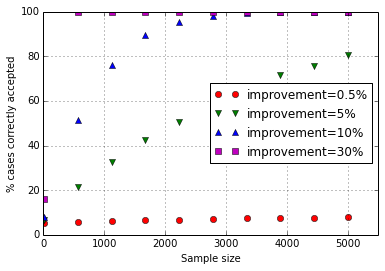As you can see from this plot, for a given sample size you are more likely to correctly decide to switch to the new webpage for larger improvements. For increases in conversion rate of 10% or more you can see that you do not need a sample with more than 2000 observations or so to gurantee you will decide to switch if there is an effect. For very small improvements you see that you need very large samples to be sure to actually detect the small improvement.

Now you know about hypothesis testing, p-values and how to use them to decide if you should switch, and you know that p-values are not all there is. The power of your test, the probability to actually detect an improvement if it is there is just as important as p-values. The beauty is that you can calculate a lot of these numbers before you ever start running an A/B test or the likes.

This post started life as a ipython notebook, download it or view it online.